• ## 时间序列分析

万次阅读 多人点赞 2017-03-22 17:04:51
http://blog.csdn.net/pipisorry/article/details/62053938时间序列简介时间序列是时间间隔不变的情况下收集的时间点集合。这些集合被分析用来了解长期发展趋势，为了预测未来或者表现分析的其他形式。但是什么时间...
http://blog.csdn.net/pipisorry/article/details/62053938时间序列简介时间序列是时间间隔不变的情况下收集的时间点集合。这些集合被分析用来了解长期发展趋势，为了预测未来或者表现分析的其他形式。但是什么时间序列？与常见的回归问题的不同？1、时间序列是跟时间有关的。所以基于线性回归模型的假设：观察结果是独立的。在这种情况下是不成立的。2、随着上升或者下降的趋势，更多的时间序列出现季节性趋势的形式，如：特定时间框架的具体变化。即：如果你看到羊毛夹克的销售上升，你就一定会在冬季做更多销售。因为时间序列的固有特性，有各种不同的步骤可以对它进行分析。时间序列的数据格式[pandas时间序列分析和处理Timeseries                    ]Note: pandas时间序列series的index必须是DatetimeIndex不能是Index，也就是如果index是从dataframe列中转换来的，其类型必须是datetime类型，不能是date、object等等其它类型！否则会报错：AttributeError: 'Index' object has no attribute 'inferred_freq'。[Decomposing trend, seasonal and residual time series elements]什么导致时间序列不稳定？这儿有两个主要原因。趋势-随着时间产生不同的平均值。举例：在飞机乘客这个案例中，我们看到总体上，飞机乘客的数量是在不断增长的。季节性-特定时间框架内的变化。举例：在特定的月份购买汽车的人数会有增加的趋势，因为车价上涨或者节假日到来。模型的根本原理或者预测序列的趋势和季节性，从序列中删除这些因素，将得到一个稳定的序列。然后统计预测技术可以在这个序列上完成。最后一步是通过运用趋势和季节性限制倒回到将预测值转换成原来的区间。时间序列的稳定性如果一个时间序列的统计特征如平均数，方差随着时间保持不变，我们就可以认为它是稳定的。稳定性的确定标准是非常严格的。但是，如果时间序列随着时间产生恒定的统计特征，根据实际目的我们可以假设该序列是稳定的。如下：恒定的平均数恒定的方差不随时间变化的自协方差为什么时间序列的稳定性这么重要？大部分时间序列模型是在假设它是稳定的前提下建立的。直观地说，我们可以这样认为，如果一个时间序列随着时间产生特定的行为，就有很高的可能性认为它在未来的行为是一样的。同时，根据稳定序列得出的理论是更加成熟的， 也是更容易实现与非稳定序列的比较。时间序列的稳定性测试1、绘制滚动统计：我们可以绘制移动平均数和移动方差，观察它是否随着时间变化。随着移动平均数和方差的变化，我认为在任何“t”瞬间，我们都可以获得去年的移动平均数和方差。如：上一个12个月份。但是，这更多的是一种视觉技术。2、DF检验：这是一种检查数据稳定性的统计测试。无效假设：时间序列是不稳定的。测试结果由测试统计量和一些置信区间的临界值组成。如果“测试统计量”少于“临界值”，我们可以拒绝无效假设，并认为序列是稳定的。[假设检验-t检验和Augmented Dickey–Fuller test                    ]from statsmodels.tsa.stattools import adfuller
def test_stationarity(timeseries):

#Determing rolling statistics
rolmean = pd.rolling_mean(timeseries, window=12)
rolstd = pd.rolling_std(timeseries, window=12)

#Plot rolling statistics:
orig = plt.plot(timeseries, color='blue',label='Original')
mean = plt.plot(rolmean, color='red', label='Rolling Mean')
std = plt.plot(rolstd, color='black', label = 'Rolling Std')
plt.legend(loc='best')
plt.title('Rolling Mean & Standard Deviation')
plt.show(block=False)

#Perform Dickey-Fuller test:
print 'Results of Dickey-Fuller Test:'
dfoutput = pd.Series(dftest[0:4], index=['Test Statistic','p-value','#Lags Used','Number of Observations Used'])
for key,value in dftest.items():
dfoutput['Critical Value (%s)'%key] = value
print dfoutputThe code is pretty straight forward. Please feel free to discuss the code in comments if you face challenges in grasping it.Let’s run it for our input series:test_stationarity(ts)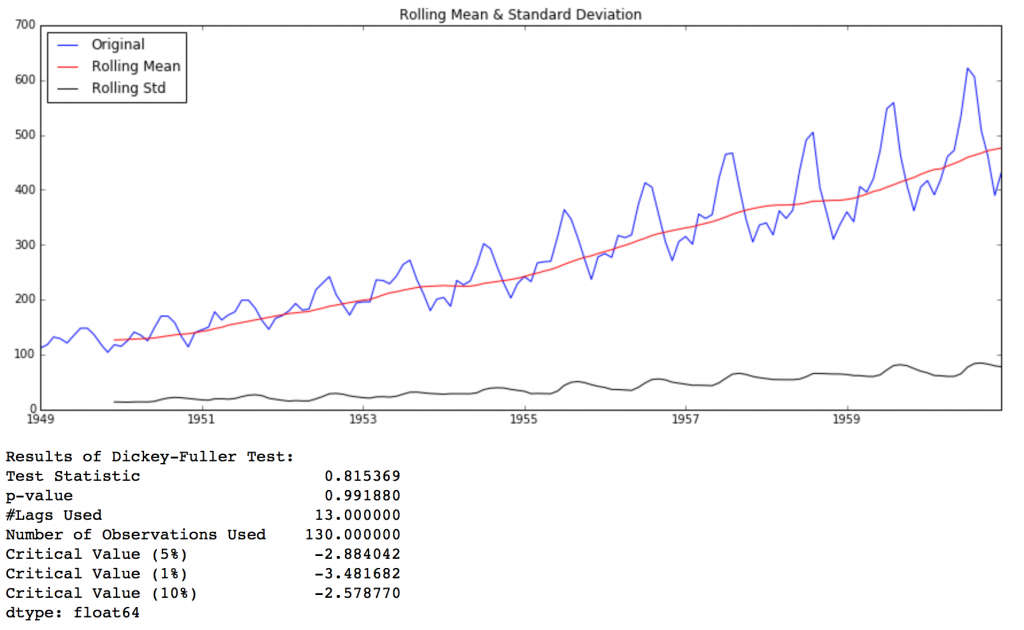皮皮blog趋势减少技术消除趋势的第一个方法是转换。例如,在本例中,我们可以清楚地看到,有一个显著的趋势。所以我们可以通过变换，惩罚较高值而不是较小值。这可以采用log,平方根,立方跟等等。ts_log = np.log(ts)我们可以使用一些技术来估计或对这个趋势建模,然后将它从序列中删除：聚合Aggregation – taking average for a time period like monthly/weekly averages取一段时间的平均值（月/周平均值）平滑Smoothing – taking rolling averages取滚动平均数多项式回归分析Polynomial Fitting – fit a regression model适合的回归模型平滑移动平均（英语：Moving Average，MA），又称“移动平均线”（简称均线），也称为滚动平均数（Rolling Average）、滚动平均值或运行平均值，是技术分析中一种分析时间序列数据的工具。移动平均可抚平短期波动，反映出长期趋势或周期。数学上，移动平均可视为一种卷积。最常见的是利用股价、回报或交易量等变数计算出移动平均。简单移动平均（英语：Simple Moving Average，SMA）是某变数之前n个数值的未作加权算术平均。例如，收市价的10日简单移动平均指之前10日收市价的平均数。加权移动平均（英语：Weighted Moving Average，WMA）指计算平均值时将个别数据乘以不同数值，在技术分析中，n日WMA的最近期一个数值乘以n、次近的乘以n-1，如此类推，一直到0。指数移动平均（英语：Exponential Moving Average，EMA或EWMA）是以指数式递减加权的移动平均。各数值的加权影响力随时间而指数式递减，越近期的数据加权影响力越重，但较旧的数据也给予一定的加权值。加权的程度以常数α决定，α数值介乎0至1。一般形式α可能的取值方法有com :     Specify decay in terms of center of mass, \alpha = 1 / (1 + com),  for com >= 0span :    Specify decay in terms of span, \alpha = 2 / (span + 1), for span > 1halflife : 使用参数“半衰期”来定义指数衰减量Specify decay in terms of half-life, \alpha = 1 - exp(log(0.5) / halflife), for halflife > 0   lz感觉0.5应该改成2？？或者pandas内部有相应的转换？span: α用天数N来代表：，所以，N=19天，代表α=0.1halflife : 在使用halflife计算移动平均时候，EMA计算中(1-α)的指数项为负数，表示与当前时间t的差值？如计算2017.2.30的EMA时，2017.2.29的指数项为-1。而在2017.2.15时，如果halflife设置为15，则p_{2015.2.15}的权重为(1-α)^-15 = (exp(log(0.5) / 15)^-15 = (exp(log(0.5) / 15 ^-15) = exp(-log0.5) = 2?[wikipedia移动平均]指数衰减某个量的下降速度和它的值成比例，称之为服从指数衰减。用符号可以表达为以下微分方程，其中N是指量，λ指衰减常数（或称衰变常数）。方程的一个解为：平均寿命如果这个衰减量是一个集合中的离散元素，可以计算元素留在集合中的平均时间长度。这被称为平均寿命（一般称寿命）。并且它可以被证明与衰减速率有关。平均时间（或被称为指数时间常数）由此被看做一个简单的缩放时间：因而，这是量减少到初始量的1/e所需要的时间。类似的，下面所述的以2为底的指数缩放时间为半衰期半衰期对多数人而言更加直观的一个典型指数衰减是当量减少为初始量的一半所需要的时间。这个时间被称为半衰期，表示为。半衰期可以被写作衰减常数或者平均寿命的形式：代入平均寿命等于半衰期除以ln2，或：[wikipedia指数衰减]皮皮blogpandas实现简单移动平均rolling这个方法有一个缺陷：要严格定义时段。在这种情况下，我们可以采用年平均数，但是对于复杂的情况的像预测股票价格，是很难得到一个数字的。所以，我们采取“加权移动平均法”可以对最近的值赋予更高的权重。Note: 前面window个rolling值是Nan，我们要丢掉这些NaN值。Series.rolling(window, min_periods=None, freq=None, center=False, win_type=None, on=None, axis=0)主要参数window : int, or offset    Size of the moving window. This is the number of observations used for calculating the statistic. Each window will be a fixed size.    If its an offset then this will be the time period of each window. Each window will be a variable sized based on the observations included in the time-period. This is only valid for datetimelike indexes.min_periods : int, default None    Minimum number of observations in window required to have a value (otherwise result is NA). For a window that is specified by an offset, this will default to 1.center : boolean, default False    Set the labels at the center of the window. By default, the result is set to the right edge of the window. This can be changed to the center of the window by setting center=True.也就是rolling方法默认是将如2011.2.1 ～ 2011.2.30的均值设置为2011.2.30的均值，center=True则是将2011.2.1 ～ 2011.2.30的均值设置为2011.2.15对应的均值。相当于将rolling线向前移动了window/2个单位。win_type : string, default None lz猜测应该是取变长window的意思，没用过。这样的话时间间隔长的timeseries的window可能小点。    Provide a window type. See the notes below.on : string, optional    For a DataFrame, column on which to calculate the rolling window, rather than the index.[ref & Examples pandas.Series.rolling]指数移动平均ewmNote: （不同于rolling）在这种情况下就不会有遗漏值因为所有的值在一开始就被赋予了权重。所以在运行的时候，它没有先前的值参与。Series.ewm(com=None, span=None, halflife=None, alpha=None, min_periods=0, freq=None, adjust=True, ignore_na=False, axis=0)主要参数com : float, optional    Specify decay in terms of center of mass, \alpha = 1 / (1 + com),  for com >= 0span : float, optional    Specify decay in terms of span, \alpha = 2 / (span + 1), for span > 1halflife : float, optional    Specify decay in terms of half-life, \alpha = 1 - exp(log(0.5) / halflife), for halflife > 0alpha : float, optional    Specify smoothing factor \alpha directly, 0 < \alpha <= 1[pandas.Series.ewm]皮皮blog趋势和季节性消除技术差分Differencing – taking the differece with a particular time lag采用一个特定时间差的差值分解Decomposition – modeling both trend and seasonality and removing them from the model.建立有关趋势和季节性的模型和从模型中删除它们差分Differencing处理趋势和季节性的最常见的方法之一就是差分法。在这种方法中,我们采用特定瞬间和它前一个瞬间的不同的观察结果。这主要是在提高平稳性。一般可以很大程度上减少了趋势。同样可以采取二阶或三阶差分在具体应用中获得更好的结果。前向差分前向差分有时候也称作数列的二项式变换函数的前向差分通常简称为函数的差分。对于函数，如果在等距节点：则称，函数在每个小区间上的增量为一阶差分。在微积分学中的有限差分（finite differences），前向差分通常是微分在离散的函数中的等效运算。差分方程的解法也与微分方程的解法相似。当是多项式时，前向差分为Delta算子（称为差分算子），一种线性算子。前向差分会将多项式阶数降低 1。逆向差分对于函数，如果：则称为的一阶逆向差分。差分的阶一阶差分的差分为二阶差分，二阶差分的差分为三阶差分，其余类推。记：为的阶差分。如果根据数学归纳法，有其中，为二项式系数。特别的，有[wikipedia差分]与差分相关的均差参考[数据插值方法]Pandas实现Series.shift(periods=1, freq=None, axis=0)[pandas.Series.shift]shift示例se
se.shift()2013-01-01    02013-01-02    12013-01-03    22013-01-04    52013-01-05    42013-01-01    NaN2013-01-02    0.02013-01-03    1.02013-01-04    2.02013-01-05    5.0pandas实现一阶差(前向差分)示例ts_diff = ts - ts.shift()分解：趋势+季节性+残差在这种方法中,趋势和季节性是分别建模的并倒回到序列的保留部分。from statsmodels.tsa.seasonal import seasonal_decompose
decomposition = seasonal_decompose(ts_log)

trend = decomposition.trend
seasonal = decomposition.seasonal
residual = decomposition.resid

plt.subplot(411)
plt.plot(ts_log, label='Original')
plt.legend(loc='best')
plt.subplot(412)
plt.plot(trend, label='Trend')
plt.legend(loc='best')
plt.subplot(413)
plt.plot(seasonal,label='Seasonality')
plt.legend(loc='best')
plt.subplot(414)
plt.plot(residual, label='Residuals')
plt.legend(loc='best')
plt.tight_layout()在这里我们可以看到趋势,季节性从数据分离，我们可以建立残差的模型，让我们检查残差的稳定性。ts_log_decompose = residual
ts_log_decompose.dropna(inplace=True)
test_stationarity(ts_log_decompose)这样时间序列是非常接近稳定。你也可以尝试高级的分解技术产生更好的结果。同时,你应该注意到, 在这种情况下将残差转换为原始值对未来数据不是很直观。[Source code for statsmodels.tsa.seasonal]Note: decomposition = seasonal_decompose(ts_log)1 如果你的数据的index不是DatetimeIndex而是其它的就会出错AttributeError: 'Index' object has no attribute 'inferred_freq'AttributeError: 'Float64Index' object has no attribute 'inferred_freq'2 如果你的数据的DatetimeIndex不能自动识别出datetime的freq就会出错ValueError: You must specify a freq or x must be a pandas object with a timeseries index这时应该手动指定decomposition = seasonal_decompose(ts_log, freq = 1)3 warnningVisibleDeprecationWarning: using a non-integer number instead of an integer will result in an error in the future皮皮blog时间序列预测从上我们看到了不同的技术，并且在使得时间序列得以稳定上运作良好。让我们通过差分后建立时间序列模型，因为差分是很受欢迎的技术，也相对更容易添加噪音和季节性从而倒回到预测残差residuals。在执行趋势和季节性评估技术上,有两种情况：值之间不含依赖的严格稳定系列。简单的情况下,我们可以建立残差residuals模型作为白噪音（指功率谱密度在整个频域内均匀分布的噪声），但这非常罕见。序列值之间含有明显依赖。在这种情况下,我们需要使用一些统计模型像ARIMA（差分自回归移动平均模型）来预测数据。这里简要介绍一下ARIMA，但不会介绍技术细节,但如果你希望更有效地应用它们，你应该理解这些概念的细节。ARIMA代表差分自回归移动平均(Auto-Regressive Integrated Moving Averages)。平稳时间序列的ARIMA预测的只不过是一个线性方程(如线性回归)。预测依赖于ARIMA模型参数(p d q)自回归项的数目Number of AR (Auto-Regressive) terms (p)：AR条件仅仅是因变量的滞后。如：如果P等于5，那么预测x（t）将是x（t-1）。。。（t-5）。移动平均项的数目Number of MA (Moving Average) terms (q):MA条件是预测方程的滞后预测错误。如：如果q等于5，预测x（t）将是e（t-1）。。。e(t-5),e(i)是移动平均叔在第ith个瞬间和实际值的差值。差分数目Number of Differences (d)：它们是非季节性的差值的数目，即这种情况下我们采用一阶差分。所以传递变量令d=0或者传递原始变量令d=1，两种方法得到的结果一样。如何确定“p”和“q”的值我们使用两个坐标来确定这些数字。自相关函数Autocorrelation Function (ACF)：这是时间序列和它自身滞后版本之间的相关性的测试。比如在自相关函数可以比较时间的瞬间‘t1’…’t2’以及序列的瞬间‘t1-5’…’t2-5’ (t1-5和t2 是结束点)。部分自相关函数Partial Autocorrelation Function (PACF):这是时间序列和它自身滞后版本之间的相关性测试，但是是在预测（已经通过比较干预得到解释）的变量后。如：滞后值为5，它将检查相关性，但是会删除从滞后值1到4得到的结果。时间序列（在差分后）的自回归函数和部分自回归函数绘制为:#ACF and PACF plots:
from statsmodels.tsa.stattools import acf, pacflag_acf = acf(ts_log_diff, nlags=20)
lag_pacf = pacf(ts_log_diff, nlags=20, method='ols')#Plot ACF:
plt.subplot(121)
plt.plot(lag_acf)
plt.axhline(y=0,linestyle='--',color='gray')
plt.axhline(y=-1.96/np.sqrt(len(ts_log_diff)),linestyle='--',color='gray')
plt.axhline(y=1.96/np.sqrt(len(ts_log_diff)),linestyle='--',color='gray')
plt.title('Autocorrelation Function')#Plot PACF:
plt.subplot(122)
plt.plot(lag_pacf)
plt.axhline(y=0,linestyle='--',color='gray')
plt.axhline(y=-1.96/np.sqrt(len(ts_log_diff)),linestyle='--',color='gray')
plt.axhline(y=1.96/np.sqrt(len(ts_log_diff)),linestyle='--',color='gray')
plt.title('Partial Autocorrelation Function')
plt.tight_layout()此图中,0每一边的两条虚线之间是置信区间。这些可以用来确定“p”和“q”的值:1、p-部分自相关函数图第一次截断上层置信区间的滞后值。如果你仔细看，该值是p=2。2、q- 自相关函数图第一次截断上层置信区间的滞后值。如果你仔细看，该值是q=2。[http://discuss.analyticsvidhya.com/t/seasonal-parameter-in-arima-and-adf-test/7385/1]现在，考虑个体以及组合效应建立3个不同的ARIMA模型。我也会打印各自的RSS（RSS表示一组统计数据的平方和的平方根）。请注意,这里的RSS是对残差值来说的，而不是实际序列。首先，我们需要ARIMA模型。from statsmodels.tsa.arima_model import ARIMAp,d,q值可以通过ARIMA的order参数即元组(p,d,q)指定。建立三种情况下的模型：自回归（AR）模型：model = ARIMA(ts_log, order=(2, 1, 0))
results_AR = model.fit(disp=-1)
plt.plot(ts_log_diff)
plt.plot(results_AR.fittedvalues, color='red')
plt.title('RSS: %.4f'% sum((results_AR.fittedvalues-ts_log_diff)**2))移动平均数（MA ）模型model = ARIMA(ts_log, order=(0, 1, 2))
results_MA = model.fit(disp=-1)
plt.plot(ts_log_diff)
plt.plot(results_MA.fittedvalues, color='red')
plt.title('RSS: %.4f'% sum((results_MA.fittedvalues-ts_log_diff)**2))组合模型model = ARIMA(ts_log, order=(2, 1, 2))
results_ARIMA = model.fit(disp=-1)
plt.plot(ts_log_diff)
plt.plot(results_ARIMA.fittedvalues, color='red')
plt.title('RSS: %.4f'% sum((results_ARIMA.fittedvalues-ts_log_diff)**2))Note: 如果报错TypeError: Cannot cast ufunc subtract output from dtype('float64') to dtype('int64') with casting rule 'same_kind'，说明时序数据的数据类型不是float类型，astype转换一下就可以了。ARMAResults及其方法[ARMAResults (class in statsmodels.tsa.arima_model)]在这里我们可以看到,自回归函数模型和移动平均数模型几乎有相同的RSS，但相结合效果显著更好。倒回到原始区间现在,我们只剩下最后一步,即把这些值倒回到原始区间。既然组合模型获得更好的结果，让我们将它倒回原始值，看看它如何执行。第一步是作为一个独立的序列，存储预测结果并观察。predictions_ARIMA_diff = pd.Series(results_ARIMA.fittedvalues, copy=True)
print predictions_ARIMA_diff.head()Note: 1 这些是从‘1949-02-01’开始,而不是第一个月。为什么?这是因为我们将第一个月份取为滞后值，一月前面没有可以减去的元素。2 这里fittedvalues是已知的数据（或者说是训练数据吧），和[statsmodels.tsa.arima_model.ARMAResults.predict]可以得到相同结果，但是不能是未来的结果。3 这里只是预测的已有的数据，实际中我们当然是要预测未来的数据。这时应该使用forecast方法，如预测最后时间之后14天的结果：print(results_ARIMA.forecast(14))[statsmodels.tsa.arima_model.ARMAResults.forecast]将差分转换为对数尺度的方法是这些差值连续地添加到基本值。一个简单的方法就是首先确定索引的累计总和,然后将其添加到基本值（这里就是第一个月的值）。predictions_ARIMA_diff_cumsum = predictions_ARIMA_diff.cumsum()
print predictions_ARIMA_diff_cumsum.head()你可以在head()使用之前的输出结果进行回算，检查这些是否正确的。接下来我们将它们添加到基本值。为此我们将使用基本值作为所有的值来创建一个序列，并添加差值。predictions_ARIMA_log = pd.Series(ts_log.ix, index=ts_log.index)
predictions_ARIMA_log.head()第一个元素是基本值本身，从基本值开始值累计添加。最后一步是将指数与原序列比较。predictions_ARIMA = np.exp(predictions_ARIMA_log)
plt.plot(ts)
plt.plot(predictions_ARIMA)
plt.title('RMSE: %.4f'% np.sqrt(sum((predictions_ARIMA-ts)**2)/len(ts)))最后我们获得一个原始区间的预测结果。虽然不是一个很好的预测。但是你获得了思路不是吗?现在,我把它留个你去进一步改进，做一个更好的方案。在本文中,我试图提供你们一个标准方法去解决时间序列问题。这里我们广泛的讨论了稳定性的概念和最终的预测残差。这是一个漫长的过程,我跳过了一些统计细节,我鼓励大家使用这些作为参考材料。皮皮blogfrom: http://blog.csdn.net/pipisorry/article/details/62053938ref: [Complete guide to create a Time Series Forecast (with Codes in Python)]*[时间序列预测全攻略][平稳时间序列预测法][时间序列分析课件 第三章 平稳时间序列分析.ppt]
展开全文• ：该方法在一个统一的框架下适用与所有的时间序列   缺点 ：模型形成需要基于知识和经验判定   平滑法应用场景  滑动平均法    霍尔特指数平滑法  霍尔特-温特指数平滑法 ...


2019独角兽企业重金招聘Python工程师标准>>>#平滑法
#滑动平均法
#霍尔特指数平滑法
#霍尔特-温特指数平滑法
#平滑法应用场景
#平滑法之移动平均法
#简单移动平滑（Single Moving Average）
#指数平滑（Exponential Moving Average）
#简单的移动平滑和指数平滑法案例
#平滑法之霍尔特指数平滑法
#平滑法之霍尔特温特指数平滑法
#霍尔特温特指数平滑法案例
#ARMA(ARIMA)法

附件是数据

平滑法
滑动平均法
处于恒定水平和没有季节性变动的时间序列

霍尔特指数平滑法
有趋势性但没有季节性因素的时间序列

霍尔特-温特指数平滑法
有趋势性且有季节性变动趋势的时间序列

ARMA(ARIMA)法
理论上可以针对数据产生的机理构建动态模型，实际上是根据数据扰动项之间相关性结构构建预测模型
优点：该方法在一个统一的框架下适用与所有的时间序列
缺点：模型形成需要基于知识和经验判定

平滑法应用场景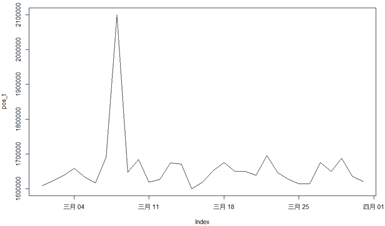滑动平均法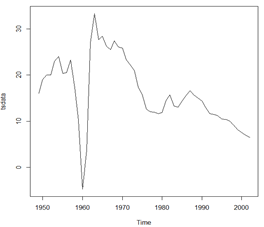霍尔特指数平滑法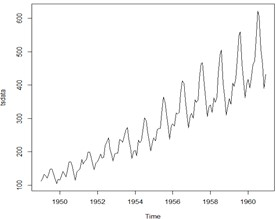霍尔特-温特指数平滑法

平滑法之移动平均法

简单移动平滑（Single Moving Average）
简单移动平均认为最近 N 期的数据对当前数据有预测作用，而且认为这 N 期数据的影响大小是相等的

数学表达式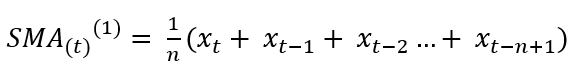n表示移动平均的期数，n越长，拟合曲线越平滑；n越短，对近期变化越敏感

指数平滑（Exponential Moving Average）
指数平滑认为最近各期的影响权重会随着时间间隔的变大而呈现指数衰减

数学表达式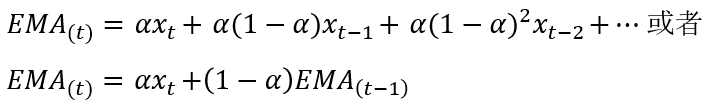平滑系数α设定较大时，近期数据的权重变大，适合预测对近期敏感的时间序列数据，而α设定较小时则适合变化缓慢的时间序列数据， α的经验值为0.05到0.3之间

简单的移动平滑和指数平滑法案例
#简单的移动平滑和指数平滑法
#股票预测案例

> library(TTR)
> data(ttrc)#股票数据

#SMA() 表示简单的移动平滑
> SMA(1:5,n=3)  # n=3 表示平均移动的期数3
 NA NA  2  3  4

#EMA() 表示指数平滑
> EMA(1:5,n=3,ratio = 0.2) # ratio表示系数α，例如最后一个预测数据根据公式等于2.92 = 5.0*0.2+2.40*2.92
   NA   NA 2.00 2.40 2.92

> sma.20 <-SMA(ttrc[,"Close"], 20) #去前面的20期做平滑统计
 51.6855 51.6930 51.7640 51.8470 51.8945 51.9440
> plot.ts(sma.20)  #如下图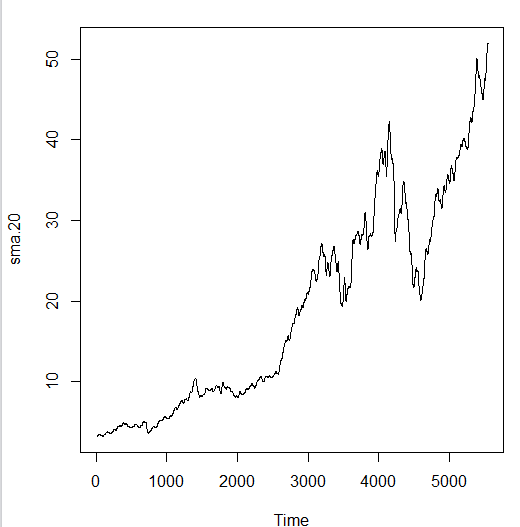平滑法之霍尔特指数平滑法
当 不考虑周期性时，并且当数据有 向上或向下的线性趋势时，可以使用 霍尔特指数平滑法（Holt Exponential Moving Average）进行预测

这种方法是指数平滑方法中的一种，其认为序列是存在一个较固定的趋势γ，那么第 t 期的估计值应该为第 t-1 期的观测值加上固定趋势的影响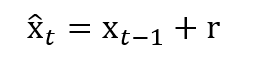每一期的固定趋势会受到随机因素的影响不恒等于r，所以r本身是一个随机序列：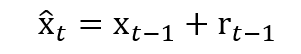加入平滑系数 ? 得到第 t 期的平滑值为：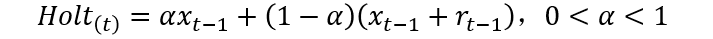由于r本身是一个随机序列，自然也可以被平滑：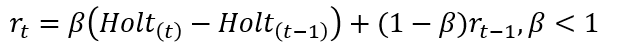?_?称为趋势序列，所以霍尔特指数平滑法是含有两个参数α和β的平滑方法

平滑法之霍尔特温特指数平滑法
当时间序列 除了趋势性之外，还表现出 明显的周期性或季节性时，可以使用 霍尔特温特指数平滑法(HoltWinters)进行预

HoltWinters来源于Holt指数平滑法，在其基础上增加了季节项，而季节效应可分为累加与累积两种

累加公式如下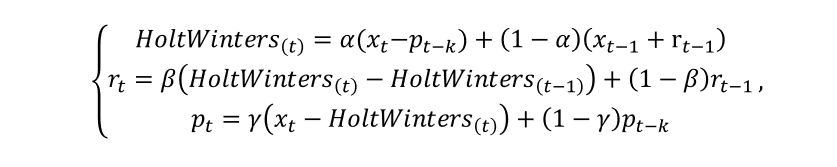累积公式如下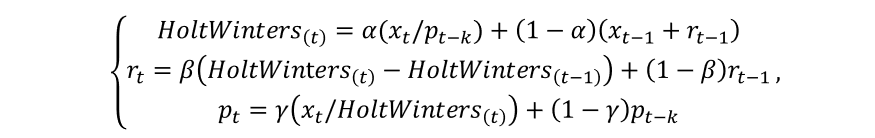两者比较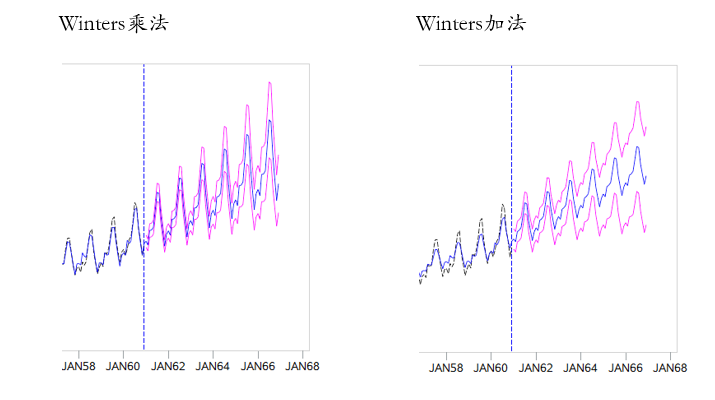霍尔特温特指数平滑法案例
#方式一

> par(mfrow = c(2,1))
> tsHW <- HoltWinters(tsdata)#霍尔特温特指数平滑法默认是累加的
> plot(tsHW) #下图中上部分
> tsHW <- HoltWinters(tsdata,seasonal = 'multi') #默认是累加的，这里用累乘的方法
> plot(tsHW) #下图中下部分，红色线是预测值，蓝色是置信区间
> tsHW.Pred<-predict(tsHW,12, prediction.interval = TRUE) #模型进行预测 predict()等于调用predict.HolWinters()进行预测
#prediction.interval = TRUE 给出预测区
#12表示预测 12个月
> tsHW.Pred
fit      upr      lwr
Jan 1961 447.0559 466.8057 427.3061
Feb 1961 419.7123 440.2920 399.1326
Mar 1961 464.8671 486.7712 442.9630
Apr 1961 496.0839 519.3350 472.8329
May 1961 507.5326 531.9278 483.1375
Jun 1961 575.4509 602.1935 548.7083
Jul 1961 666.5923 696.5558 636.6288
Aug 1961 657.9137 688.6454 627.1821
Sep 1961 550.3088 578.9777 521.6398
Oct 1961 492.9853 520.9553 465.0153
Nov 1961 420.2073 446.9458 393.4688
Dec 1961 465.6345 487.9686 443.3004
> plot(tsHW,tsHW.Pred)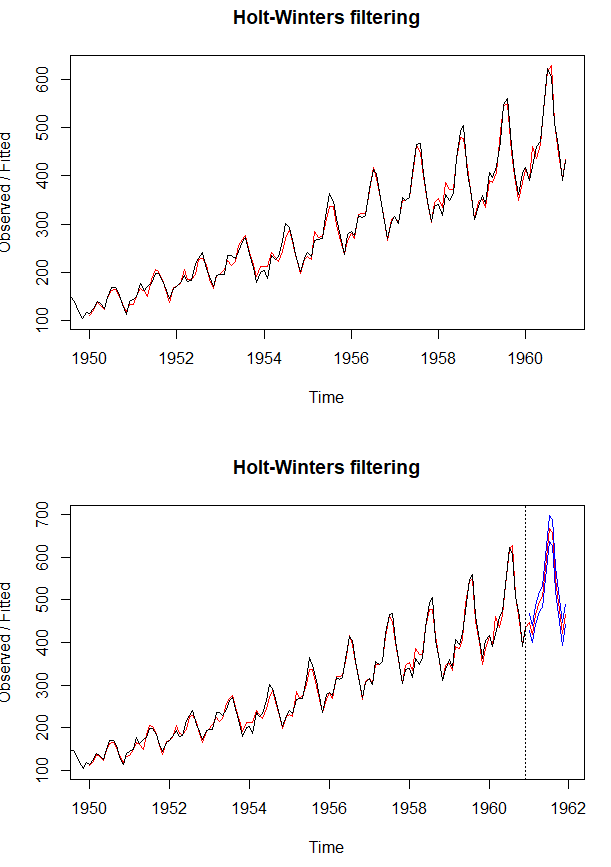#方式二：使用 forecast包中的 hw方法()

> library(forecast)
> par(mfrow = c(2,1))
> plot(hw(tsdata,h = 12))  #如下图上部分
> plot(hw(tsdata,seasonal='multi',h = 12)) #如下图下部分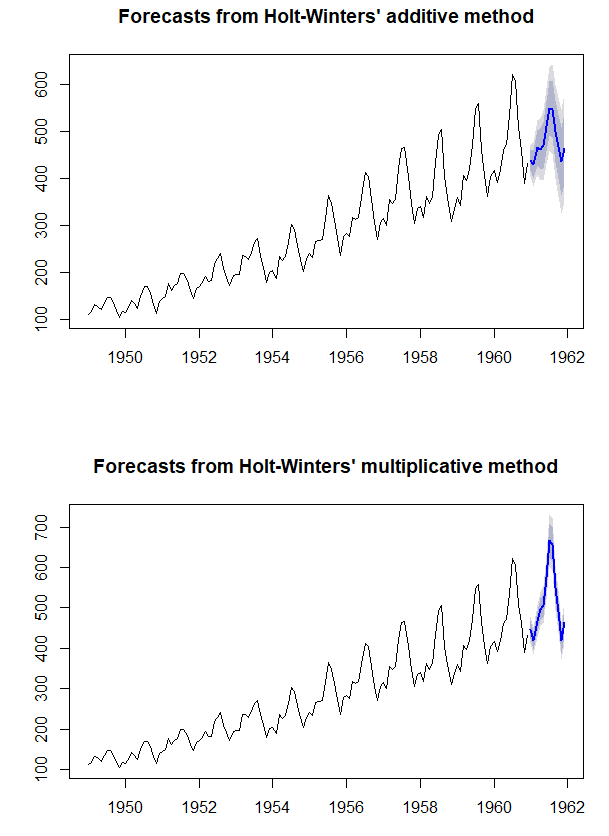转载于:https://my.oschina.net/u/1785519/blog/1572616
展开全文• ## 时间序列分析基础

千次阅读 2016-01-05 12:55:01
时间序列是现实生活中经常会碰到的数据形式。...由于工作需要，我最近简单学习了时间序列分析相关的基础理论和应用方法，这篇文章可以看做是我的学习笔记。 文章主要内容会首先描述时间序列分析的基本概念和相关

时间序列是现实生活中经常会碰到的数据形式。例如北京市连续一年的日平均气温、某股票的股票价格、淘宝上某件商品的日销售件数等等。时间序列分析的的目的是挖掘时间序列中隐含的信息与模式，并借此对此序列数据进行评估以及对系列的后续走势进行预测。

由于工作需要，我最近简单学习了时间序列分析相关的基础理论和应用方法，这篇文章可以看做是我的学习笔记。

文章主要内容会首先描述时间序列分析的基本概念和相关的统计学基础理论，然后着重讲述十分经典和常用的ARIMA模型，在这之后会讲述季节ARIMA模型。

由于打算以学习笔记的形式写这篇文章，所以我不会一下子写完整篇文章才发布，而是持续更新这篇文章，写的过程中也可能会对前面的内容进行修订。

文章中会穿插许多实例，分析过程中将使用R为分析工具。

基本概念

时间序列

简单来说，时间序列是一个变量在不同时间点的值所组成的有序序列。例如北京市2013年4月每日的平均气温就构成了一个30个元素的时间序列，为了方便，我们一般认为序列中相邻元素具有相同的时间间隔。

时间序列可以分为确定的和随机的。例如一个1990年出生的人，从1990年到1999年年龄可以表述为{0,1,2,…,9}{0,1,2,…,9}，这个序列并没有任何随机因素。不过现实生活中我们面对的更多的是掺杂了随机因素的时间序列，例如气温、销售量等等。

时间序列分析

时间序列分析说白了就是寻找时间序列中的模式。如果是在确定性时间序列中，这就基本等价于寻找序列的通项公式，例如上面年龄的时间序列，用差为1的等差数列公式就可以很好的描述其模式。

当然实际的时间序列分析基本都是针对随机时间序列。对于随机时间序列，情况会复杂一些，但本质上还是可以看做寻找通项公式（可以是封闭形式或递推形式），只不过我们面对的序列存在随机扰动，所以分析过程中除了确定性序列分析的技术外，还需要一些概率统计方面的知识和方法，下面一节会介绍一些相关的基础知识。

主要统计量

注意时间序列中的每一个元素都是一个普通的随机变量，如果忽略序列的时间性，那么我们面对的实际上是一个随机变量集合，所以从这个角度来说时间序列的统计分析与普通统计分析没有太大不同，相关的理论也是通用的。

对于随机变量集合来说，要完整描述其统计特性需要处理其多元联合分布，这是非常复杂的。所以实际我们往往做一些必要的简化假设，避免处理复杂的多元联合分布。

现假设我们有随机时间序列

{Yt|t=0,±1,±2,⋯}{Yt|t=0,±1,±2,⋯}

下面先给出一些常用的统计量。后面会接着通过一些常见序列来举例说明各统计量如何计算。

均值

均值函数被定义为关于自变量t的函数：

μt=E(Yt)μt=E(Yt)

t的均值函数值表示在t时刻随机变量YtYt的期望。

方差

与均值类似，方差是t时刻序列元素的方差：

σ2t=E((Yt−μt)2)σt2=E((Yt−μt)2)

自协方差

自协方差是一个二元函数，其自变量为两个时间点，值是两个时间点上序列值的协方差：

γt,s=Cov(Yt,Ys)=E((Yt−μt)(Ys−μs))γt,s=Cov(Yt,Ys)=E((Yt−μt)(Ys−μs))

当t=s时，自协方差就是t时刻的方差。

自相关系数

自相关系数是两个时刻的值的相关系数：

ρt,s=γt,sγt,tγs,s−−−−−√ρt,s=γt,sγt,tγs,s

如果忽略元素来自时间序列这一事实，各统计量的意义实际上与普通的统计学中无异。因此这些统计量的一些性质也可以无缝推广到时间序列分析。例如期望的线性性质等等。如果有需要可以自行复习一下这些统计量的相关计算性质。后面的推导会主要集中于这几个统计量的计算。

时间序列示例

下面看几个简单的随机时间序列示例。

白噪声

考虑一个时间序列，其中每一个元素为独立同分布变量，且均值为0。这种时间序列叫做白噪声。之所以叫这个名字，是因为对这种序列的频域分析表明其中平等的包含了各个频率，和物理中的白光类似。

下面是用R模拟生成的白噪声时序图。Y = ts(rnorm(100, mean=0, sd=1));plot(Y, family="simhei", main="白噪声", type="b", col="red");abline(h=0)

其中共100个元素，每个元素都独立服从标准正态分布N(0,1)N(0,1)。可以从图中看出白噪声基本是在均值附近较为平均的随机震荡。

由于每个元素服从N(0,1)N(0,1)，所以均值μt=0μt=0，方差σ2t=1σt2=1。又因为每个元素独立，所以对于任何t≠st≠s，γt,s=0γt,s=0，ρt,s=0ρt,s=0。这些统计特征与对图像的直观观察基本一致。

白噪声的重要之处在于很多其它的重要时间序列都可以通过它构造出来，这一点下文会看到。我们一般用e表示白噪声，将白噪声序列写作：

{e1,e2,…,et,…}{e1,e2,…,et,…}

随机游走

下面考虑这样一个时间序列，其在t时刻的值是前面白噪声序列的前t个值之和，设{e1,e2,…,et,…}{e1,e2,…,et,…}为标准正态分布产生的白噪声，则：

Y1Y2Yt==⋮=⋮e1e1+e2e1+e2+⋯+etY1=e1Y2=e1+e2⋮Yt=e1+e2+⋯+et⋮

下面是用R模拟的随机游走。Y = ts(rnorm(100, mean=0, sd=1));for (i in 2:length(Y)) {    Y[i] = Y[i] + Y[i-1];}plot(Y, family="simhei", main="随机游走", type="b", col="red");abline(h=0)

可以看到随机游走比白噪声平滑很多，并且呈现出一些“趋势性”的感觉。下面分析其相关统计特征。

均值：μt=E(e1+⋯+et)=E(e1)+⋯+E(et)=0μt=E(e1+⋯+et)=E(e1)+⋯+E(et)=0

方差：σ2t=Var(e1+⋯+et)=Var(e1)+⋯+Var(et)=tσ2σt2=Var(e1+⋯+et)=Var(e1)+⋯+Var(et)=tσ2

对协方差的计算需要用到一个协方差性质：

Cov(∑i=1mciYi,∑j=1ndjYj)=∑i=1m∑j=1ncidjCov(Yi,Yj)Cov(∑i=1mciYi,∑j=1ndjYj)=∑i=1m∑j=1ncidjCov(Yi,Yj)

设t小于s，由于只有i=j时Cov(Yi,Yj)=σ2Cov(Yi,Yj)=σ2，所以：

自协方差：γt,s=tσ2γt,s=tσ2

自相关系数：ρt,s=tσ2tsσ4√=ts√ρt,s=tσ2tsσ4=ts

从统计性质可以看到，随机游走的“趋势性”实际是个假象，因为其均值函数一直是白噪声的均值，不存在偏离的期望。但是方差与时间呈线性增长并且趋向于无穷大，这意味着只要时间够长，随机游走的序列值可以偏离均值任意远，但期望永远在均值处。

物理与经济学中的很多现象都被看做是随机游走，例如分子的布朗运动，股票的价格走势等等。

从协方差和相关系数看，如果起点t固定，则越接近的点相关性越大，例如ρ1,2=0.707ρ1,2=0.707，ρ1,3=0.577ρ1,3=0.577，ρ1,4=0.500ρ1,4=0.500。同时，起点不同，时滞相同自相关系数也不同，越往后同时滞自相关系数越大，例如ρ2,3=0.816ρ2,3=0.816，ρ3,4=0.866ρ3,4=0.866。

实际上从纯数学角度可以将自相关系数看成一个二元函数，自变量是时间点t和时滞s-t。认识到这点很重要，因为它与时间序列分析中一个重要的概念——平稳性有着密切的关系。

平稳性

平稳性是时间序列分析中很重要的一个概念。一般的，我们认为一个时间序列是平稳的，如果它同时满足一下两个条件：

1）均值函数是一个常数函数

2）自协方差函数只与时滞有关，与时间点无关

以上面两个时间序列为例。两个序列均满足条件1），因为标准正态分布白噪声和其形成的随机游走的均值函数都是值恒为0的常数函数。再来看条件2）。白噪声的自协方差函数可以表述为：

γt,s={10(t=s)(t≠s)γt,s={1(t=s)0(t≠s)

可以看到只有在时滞为0时值为1，其它均为0，所以白噪声是一个平稳序列。

而随机游走我们上面分析过，其自协方差为：

γt,s=tσ2γt,s=tσ2

很明显其自协方差依赖于时间点，所以是一个非平稳序列。

后面可以看到，一般的时间序列分析往往针对平稳序列，对于非平稳序列会通过某些变换将其变为平稳的，例如，对于随机游走来说，其一阶差分序列是平稳的（显然其一阶差分是白噪声）。

下一章节会介绍ARIMA模型，其中将对上面提到的平稳性和差分的概念给出更具体的说明的示例。

注意我们下面说到平稳序列时都默认其均值为0，因为具有均值μμ的平稳时间序列只要将其做一个变换Yt−μYt−μ就可以得到一个均值为0的序列，假设均值为0可以使得问题分析得到简化。

ARIMA模型

基本模型

上文说过，时间序列分析实际上是寻找随机时间序列中的模式，所以首先要对时间序列做一个假设，假设其符合某个模式。具体一点，就是时间序列可以用一个函数（可以包含随机变量）来描述。函数的自变量是时刻，值是这个时刻序列的值。例如上例中的白噪声可以用f(t)=ef(t)=e描述，其中e是一个服从标准正态分布的随机变量。

时间序列分析的核心工作之一就是根据观察到的序列值来估计这个函数。

其中ARIMA模型是一个常用的函数模型。实际上ARIMA模型本身并不是一个具体描述时间序列的函数，而是一类函数的总称。ARIMA模型可以表述为ARIMA(p,d,q)ARIMA(p,d,q)，其中p、d和q定义域均为自然数。从这个角度看，ARIMA可以看成函数的函数，或者叫做高阶函数。这个高阶函数将一个定义在自然数上的三元空间映射到一个具体函数，具体函数可以描述一个时间序列。

例如：

(ARIMA(0,0,1))(t)(ARIMA(0,0,2))(t)(ARIMA(1,0,0))(t)(ARIMA(1,0,2))(t)====et−θet−1et−θ1et−1−θ2et−2et+ϕ(ARIMA(1,0,0))(t−1)et+ϕ(ARIMA(1,0,2))(t−1)−θ1et−1−θ2et−2(ARIMA(0,0,1))(t)=et−θet−1(ARIMA(0,0,2))(t)=et−θ1et−1−θ2et−2(ARIMA(1,0,0))(t)=et+ϕ(ARIMA(1,0,0))(t−1)(ARIMA(1,0,2))(t)=et+ϕ(ARIMA(1,0,2))(t−1)−θ1et−1−θ2et−2

当不同参数取0时ARIMA可以退化为更简单的形式，例如d=0时，模型变为ARMA，如果d和q都等于0，就变为AR模型，而如果d和p为0，则是MA模型，如果d、p和q都为0，那就是白噪声了。所以ARIMA(0,0,0)ARIMA(0,0,0)就是白噪声序列函数，因此白噪声也只是ARIMA模型的一个特例。

下图说明了各个模型间的关系：图中自顶向下越来越特化，而自底向上则越来越泛化。

这一节我们从较为简单的特化ARIMA模型开始讲述，由简入难，一步一步描述各种模型。后面的几节会讲述如何对ARIMA模型进行训练和估计以及如何应用ARIMA模型进行预测。

MA模型

ARIMA中的p、d和q分别表示自相关、差分和滑动平均。当p和d均为0时，就变成了简单的滑动平均模型MA(q)MA(q)。

一般的滑动平均模型被定义为：

Yt=et−θ1et−1−θ2et−2−⋯−θqet−qYt=et−θ1et−1−θ2et−2−⋯−θqet−q

其中e是方差为σ2σ2的白噪声。并且要求各参数−θ−θ是定义在-1到1的闭区间上。

可以看出，MA(q)在t时刻的值就是白噪声序列t到t-q共q+1个点的线性组合，系数是(1,−θ1,…,−θq)(1,−θ1,…,−θq)。

简单起见，我们分析一下最简单的MA(1)和MA(2)模型及其统计性质。

下面是MA(1)模型的序列函数以及用R产生的模拟数据：

Yt=et−θet−1Yt=et−θet−1Ye = rnorm(100, mean=0, sd=1);Y = c();Y = Ye;for (i in 2:length(Ye)) {    Y[i] = Ye[i] - (-0.8) * Ye[i-1];}Y = ts(Y);plot(Y, family="simhei", main="MA(1)", type="b", col="red");abline(h=0)

这个模拟数据构造自服从标准正态分布的白噪声，其中一阶滑动参数为-0.8。从图上看，这个序列比白噪声平滑，并且比随机游走平稳一些。下面定量分析其各统计量。

均值：

μt=E(Yt)=E(et)−θE(et−1)=0μt=E(Yt)=E(et)−θE(et−1)=0

方差：

σ2t=Var(Yt)=Var(et)+θ2Var(et−1)=(1+θ2)σ2σt2=Var(Yt)=Var(et)+θ2Var(et−1)=(1+θ2)σ2

自协方差：

γt,s=Cov(et−θet−1,es−θes−1)=Cov(et,es)−θCov(et,es−1)−θCov(et−1,es)+θ2Cov(et−1,es−1)γt,s=Cov(et−θet−1,es−θes−1)=Cov(et,es)−θCov(et,es−1)−θCov(et−1,es)+θ2Cov(et−1,es−1)

显然，在t小于s时，只有s-t=1时，有Cov(et,es−1)=Var(et)=σ2Cov(et,es−1)=Var(et)=σ2。所以自协方差函数只与时滞s-t有关，我们将自协方差表示为时滞k的函数γkγk，我们有：

γk=⎧⎩⎨⎪⎪(1+θ2)σ2−θσ20(k=0)(k=1)(k>1)γk={(1+θ2)σ2(k=0)−θσ2(k=1)0(k>1)

自相关系数：

ρk=⎧⎩⎨⎪⎪1(−θ)/(1+θ2)0(k=0)(k=1)(k>1)ρk={1(k=0)(−θ)/(1+θ2)(k=1)0(k>1)

从上面分析得出，MA(1)模型是一个平稳序列，因为其均值为常数，自协方差只与时滞相关。以后任何平稳模型，我们都将自协方差和自相关系数表示为时滞k的函数，而不再表示为t和s的函数。另外还可以发现，MA(1)模型每一个序列值只与其前一个值有相关性，而时滞超过1则无相关性，后面可以看到这个特性是识别MA模型的重要特征。另外不难证明，一阶自相关系数在θθ为正负1时分别达到最强负相关-0.5和最强正相关0.5，在θθ为0时，MA(1)退化为白噪声，因此自相关系数为0。

类似的，MA(2)模型可以表述为：

Yt=et−θ1et−1−θ2et−2Yt=et−θ1et−1−θ2et−2Ye = rnorm(100, mean=0, sd=1);Y = c();Y = Ye;Y = Ye - (-0.8) * Ye;for (i in 3:length(Ye)) {    Y[i] = Ye[i] - (-0.8) * Ye[i-1] - (-0.9) * Ye[i-2];}Y = ts(Y);plot(Y, family="simhei", main="MA(2)", type="b", col="red");abline(h=0)

如果做统计分析，会发现MA(2)模型也是一个平稳模型，并且在时滞大于2后没有相关性。

一般的，MA(q)模型是一个平稳模型，并且在时滞大于q后没有相关性。此处不再给出一般MA模型的统计分析，有兴趣的朋友可以自行推导。

AR模型

现在考虑另一种模型：t时刻的序列值与其滞后p的p个时间序列呈多元线性相关：

Yt=et+ϕ1Yt−1+ϕ2Yt−2+⋯+ϕpYt−pYt=et+ϕ1Yt−1+ϕ2Yt−2+⋯+ϕpYt−p

从公式上看，AR应该比MA具有更强的自相关性，因为MA仅与滞后的白噪声因素相关，而AR是时间序列前后直接相关。由于AR模型的统计特性推导比MA复杂很多，所以我们先分析最简单的AR(1)，借此了解AR模型的特性。

AR(1)的序列公式如下：

Yt=et+ϕYt−1Yt=et+ϕYt−1

与MA(1)不同，这是一个递推公式，并且是一个线性递推公式，我们可以把它展开：

Yt=et+ϕet−1+ϕ2et−2+⋯+ϕket−k+⋯Yt=et+ϕet−1+ϕ2et−2+⋯+ϕket−k+⋯

我们会得到一个无穷级数表达式（假设原始白噪声序列有无穷多滞后项）。显然其均值为0。方差的计算如下：

σ2t=Var(Yt)=(1+ϕ2+ϕ4+⋯+ϕ2k+⋯)σ2=(limn→∞1−ϕ2n1−ϕ2)σ2σt2=Var(Yt)=(1+ϕ2+ϕ4+⋯+ϕ2k+⋯)σ2=(limn→∞1−ϕ2n1−ϕ2)σ2

显然只有当|ϕ|<1|ϕ|<1时级数收敛到σ21−ϕ2σ21−ϕ2

这里不加证明给出一个重要的结论：AR(1)是平稳的当且仅当|ϕ|<1|ϕ|<1。下面我们假设序列满足平稳条件，推导其自协方差和自相关系数。

γk=====E((Yt−k−μt−k)(Yt−μt))E(Yt−kYt)E(Yt−ket+ϕYt−kYt−1)E(Yt−k)E(et)+ϕE(Yt−kYt−1)ϕγk−1γk=E((Yt−k−μt−k)(Yt−μt))=E(Yt−kYt)=E(Yt−ket+ϕYt−kYt−1)=E(Yt−k)E(et)+ϕE(Yt−kYt−1)=ϕγk−1

由上面的递推式得：

γk=ϕkγ0=ϕkσ21−ϕ2γk=ϕkγ0=ϕkσ21−ϕ2

ρk=γk/γ0=ϕkρk=γk/γ0=ϕk

从统计特性可以知道，AR(1)模型相近的时序点倾向于一起“运动”，因此可能呈现假趋势。前置节点的影响随着时滞的增大而呈指数衰减。这种特性对于模型识别非常重要。

下面是一个R模拟的AR(1)时间序列。Ye = rnorm(100, mean=0, sd=1);Y = c();Y = Ye;for (i in 2:length(Ye)) {    Y[i] = Ye[i] + 0.8 * Y[i-1];}Y = ts(Y);plot(Y, family="simhei", main="AR(1)", type="b", col="red");abline(h=0)

下面说一下AR模型的平稳条件。为了讨论这点，我们先引进一个定义：AR模型的特征方程。一般的，AR(p)模型的特征方程被定义为：

1−ϕ1x−ϕ2x2−⋯−ϕpxp=01−ϕ1x−ϕ2x2−⋯−ϕpxp=0

显然一个AR(p)的特征方程是一个一元p次方程。

已经证明：一个AR模型是平稳的当且仅当其特征方程的所有根的绝对值大于1。

利用这个结论可以将AR的平稳性问题转化为一个代数问题。例如上面的AR(1)模型，其特征方程为1−ϕx=01−ϕx=0，唯一的根为x=1/ϕx=1/ϕ，因此AR(1)的平稳条件是|1/ϕ|>1|1/ϕ|>1，等价于|ϕ|<1|ϕ|<1，这是上面给出过的AR(1)平稳条件。

当p大于1时，因为特征方程可能存在复数根，所以平稳条件的计算涉及比较复杂的线性代数和复变函数知识，这里就不再详述了。

对于一般AR模型

Yt=et+ϕ1Yt−1+ϕ2Yt−2+⋯+ϕpYt−pYt=et+ϕ1Yt−1+ϕ2Yt−2+⋯+ϕpYt−p

假设AR已经满足平稳性条件，有如下统计特性：

对上式两边求期望，可得μ=E(Yt)=(ϕ1+⋯+ϕp)μμ=E(Yt)=(ϕ1+⋯+ϕp)μ，要使此等式恒成立显然：

μ=0μ=0

对上式两边乘以Yt−kYt−k，然后求期望，可得自协方差递推式：

γk=E(YtYt−k)=ϕ1γk−1+⋯+ϕpγk−pγk=E(YtYt−k)=ϕ1γk−1+⋯+ϕpγk−p

再除以γ0γ0自相关系数递推式：

ρk=E(YtYt−k)=ϕ1ρk−1+⋯+ϕpρk−pρk=E(YtYt−k)=ϕ1ρk−1+⋯+ϕpρk−p

而对于初始值ρ1,…,ρpρ1,…,ρp的求解，根据平稳序列自相关系数的稳定性，有ρ−k=ρkρ−k=ρk，再加上ρ0=1ρ0=1，带入上面递推式可得一个含有p个未知量的线性方程组，解方程组就可以得到ρ1,…,ρpρ1,…,ρp。

通过上面的分析可以发现AR模型统计特性的分析最终会归结为线性代数问题，不过在现实的时间序列分析中能否从数学意义上理解上述过程并不是重点，重点是直观理解AR(p)模型在不同的参数ϕϕ下其自相关系数随时滞k的变化情况。

而且现实建模时一般很少使用高于AR(2)的模型，因为过高的阶会导致复杂的模型和提高过拟合风险。因此在实际使用中了解AR(1)和AR(2)的特性一般就足够了，后面在模型识别中会结合图形描述AR(2)的自相关函数特性。

ARMA模型

如果一个时间序列兼有AR和MA部分，并且是平稳的，则构成ARMA模型。一般ARMA(p,q)ARMA(p,q)的表达式为：

Yt=et+ϕ1Yt−1+ϕ2Yt−2+⋯+ϕpYt−p−θ1et−1−θ2et−2−⋯−θqet−qYt=et+ϕ1Yt−1+ϕ2Yt−2+⋯+ϕpYt−p−θ1et−1−θ2et−2−⋯−θqet−q

已经证明，ARMA序列是平稳的当且仅当其AR特征方程的根的模大于1。因此求解ARMA平稳条件与AR平稳条件无异，只需忽略MA部分直接套用AR平稳条件求解即可。换句话说，ARMA(p,q)平稳当且仅当AR(p)平稳。

下面研究最简单的ARMA(1,1)模型。这是一个带有一阶自回归和一阶滑动平均的序列：

Yt=et+ϕYt−1−θet−1Yt=et+ϕYt−1−θet−1

其平稳的条件等于AR(1)的平稳条件，也就是|ϕ|<1|ϕ|<1。在这个前提下，我们分析其统计特性。由于推导过程比较复杂，这里直接把我之前在纸上推导的草稿贴出来，详见下图（点击图片可放大）。其中结论性部分我用红笔标出了。根据上面的推导结果，ARMA(1,1)的统计特性如下：

μ=0μ=0

γk=⎧⎩⎨⎪⎪⎪⎪⎪⎪1+θ2−2ϕθ1−ϕ2σ2ϕγ0−θσ2ϕγk−1(k=0)(k=1)(k≥2)γk={1+θ2−2ϕθ1−ϕ2σ2(k=0)ϕγ0−θσ2(k=1)ϕγk−1(k≥2)

ρk=ϕk−1(ϕ−θ)(1−ϕθ)1+θ2−2ϕθρk=ϕk−1(ϕ−θ)(1−ϕθ)1+θ2−2ϕθ

大约可以看到相关系数也是随时滞呈指数递减，当然不同的参数会有不同的情况，具体我们留待模型识别一节讨论。

下面给出一个模拟的ARMA(1,1)时间序列：Ye = rnorm(100, mean=0, sd=1);Y = c();Y = Ye;for (i in 2:length(Ye)) {    Y[i] = Ye[i] + 0.8 * Y[i-1] - (-0.9) * Ye[i-1];}Y = ts(Y);plot(Y, family="simhei", main="ARMA(1,1)", type="b", col="red");abline(h=0)

ARIMA模型

平稳性、可逆性及一般线性过程

模型识别

参数估计

模型预测

实例：使用R进行ARIMA时间序列分析

模型诊断

季节ARIMA模型
from: http://blog.codinglabs.org/articles/time-series-analysis-foundation.html

展开全文• ## 常见时间序列模型

千次阅读 2018-12-30 11:50:22
本文主要对各种时间序列模型及其特征做了一个归纳总结，以便查询了解。 符号说明： 变量： x,yx,yx,y 变量集：X,YX,YX,Y 变量xxx在ttt时刻的值：xtx_txt​ 参数：α,β\alpha, \betaα,β ##自回归模型...
本文主要对各种时间序列模型及其特征做了一个归纳总结，以便查询了解。
符号说明：
变量： $x,y$
变量集：$X,Y$
变量$x$在$t$时刻的值：$x_t$
参数：$\alpha, \beta$
自回归模型（Autoregressive model，AR）
自回归，顾名思义，就是用自己预测自己，即用同一变量$x$之前的信息$\{x_1,x_2,\dots,x_{t-1}\}$来预测$x$当前时刻$x$的信息$x_t$，并假设他们是线性关系。
定义
$X_t =\sum_{i=1}^{p}\varphi X_{t-i}+\varepsilon_{t}+c\\ =\sum_{i=1}^{p}\varphi X_{t-i}+c$
其中，$\varepsilon_{t}$是均值为0，方差为$\sigma^2$的随机误差值，假设$\sigma$对于任何的$t$都不变；$c$是常数项。
优缺点
优点：所需的数据或信息不多，只用自身来预测自身；
缺点：必须具有自相关性；只能适用于预测与自身前期相关的现象。
移动平均模型（Moving average model，MA）
移动平均模型也被称为移动平均过程，是一种常见的对单变量时间序列（univariate time series）建模的方法。它指出输出变量线性依赖于当前值和不同随机项的过去值。
定义
q阶移动平均模型通常简记为MA(q)：
$X_{t} = \varepsilon_{t}+\theta_{1}\varepsilon_{t-1}+\dots+\theta_{q}\varepsilon_{t-q}+\mu\\ =\varepsilon_{t}+\sum_{j=1}^{q}\theta_{j}\varepsilon_{t-j}+\mu$
其中，$\theta_{1},\dots,\theta_{q}$是序列的参数，$\varepsilon_{1},\dots,\varepsilon_{t-q}$是白噪声、随机误差项，$\mu$是序列的均值。
其也可以表示成：
$X_{t}=(1+\theta_{1}B+\dots+\theta_{q}B^{q})\varepsilon_{t}+\mu$
自回归滑动平均模型（Autoregressive moving average model，ARMA）
自回归滑动平均模型可以看成是由自回归模型和移动平均模型“混合”构成的弱平稳随机过程。
当系统是一系列未观察到的冲击（MA部分）以及它自己行为的函数时，使用ARMA是合适的。例如，股票价格可能会受到基本信息的冲击，以及由于市场参与者而表现出的技术趋势和均值回归效应。
定义
ARMA(p,q)表示$p$阶AR和$q$阶MA：
$X_t = \sum_{i=1}^{p}\varphi X_{t-i}+\varepsilon_{t}+\sum_{j=1}^{q}\theta_{j}\varepsilon_{t-j}+c$
差分回归移动平均模型（Autoregressive integrated moving average，ARIMA）
在统计、经济学和时间序列分析中，ARIMA模型是ARMA模型的扩展，二者都是适用于时序数据更好地理解数据和预测序列中未来的点。ARIMA可以适用于数据非稳态的情况。
定义
ARIMA(p,d,q)中，AR是"自回归"，$p$为自回归项数；MA为"滑动平均"，$q$为滑动平均项数，$d$为使之成为平稳序列所做的差分次数（阶数）。其定义为：
$\left (1-\sum_{i=1}^{p}\phi_{i}L^{i} \right)(1-L)^{d}X_{t}=\left(1+\sum_{i=1}^{q}\theta_{i}L^{i} \right)\varepsilon_{t}$
其中，$L$是时间滞后算子（lag operator），$d \in \mathcal{Z},d>0$。
矢量自回归模型（Vector autoregression，VAR）
矢量自回归模型是一个用于捕捉多元时间序列间的线性独立性的一种随机过程模型。与AR模型的区别就是其允许多于一个变量。
定义
矢量自回归模型描述了$k$个变量之间在相同的时间段内跟他们过去时刻的值的线性函数的演变。
一个$p$阶的VAR模型简写为VAR$(p)$，其定义为：
$x_t=A_1x_{t-1}+A_{2}x_{t-2}+\dots+A_{p}x_{t-p}+e_{t}+c$
其中，A_{i}表示变量不同时间之间的影响强度，是$k\times k$的矩阵；